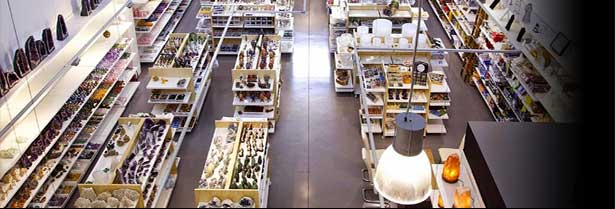### Specialized store in wholesale of mineralsQuantity:

Quantity:

Quantity:

Quantity:

Quantity:

Quantity:

Quantity:

Quantity:

Quantity:

Quantity:

Quantity:

Quantity:

Quantity:

Quantity:

Quantity:

Quantity:

Quantity:

Quantity:

Quantity:

Quantity:

Quantity:

Quantity:

Quantity:

Quantity:

Quantity:

Quantity:

Quantity:

Quantity:

Quantity:

Quantity:

Quantity:

Quantity:

Quantity:

Quantity:

Quantity:

Quantity:

Quantity:

Quantity:

Quantity:

Quantity:

Quantity:

Quantity:

Quantity:

Quantity:

Quantity:

Quantity:

Quantity:

Quantity:

Quantity:

Quantity:

Quantity:

Quantity:

Quantity:

Quantity:

Quantity:

Quantity:

Quantity:

Quantity:

Quantity:

Quantity:

Quantity:

Quantity:

Quantity:

Quantity:

Quantity:

Quantity:

Quantity:

Quantity:

Quantity:

Quantity:

Quantity:

Quantity:

Quantity:

Quantity:

Quantity:

Quantity:

Quantity:

Quantity:

Quantity:

Quantity:

Quantity:

Quantity:

Quantity:

Quantity:

Quantity:

Quantity:

Quantity:

Quantity:

Quantity:

Quantity:

Quantity:

Quantity:

Quantity:

Quantity:

Quantity:

Unavailable

Quantity:

Quantity:

Quantity: